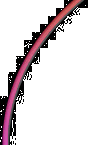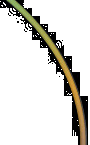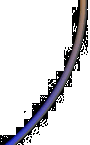# FoggyLog

## Wed Dec 29th 2004 13:09:22: dec2bin() for more than 8 bits in Excel

Well it's very very quiet here. Which is quite nice.

Today's useful piece of information is a version of DEC2BIN that works for more than 8 bits (which is the limit of Excel's built in function):

`=DEC2BIN((MOD(A1,4096)/512),3) & DEC2BIN(MOD(A1,512),9)`

This works for up to 12 bits, however the principle can probably be extended for more bits. (note that it won't complain if you pass it a number greater than 4095, it'll just loose the most significant bits).

THANK YOU !!!!!!!!!!!!!!!!!!!!!!!!!!!!!!!!!!!!!!!!!!!

Comment by Matt at 14:52 on Fri 6 October 2006

Hey! Thank you. This just saved my life!

Comment by Pedro at 13:28 on Thu 14 December 2006

this doesn't work for me...

if i copy your formula in an excel cell, the result of any number I want to convert is blank..

Comment by Niko at 9:47 on Wed 7 February 2007

Niko,

Make sure you have turned on the "Analysis ToolPak" Add In.

To do this (in Excel 2003) go to "Tools" > "Add-Ins..." and then tick "Analysis ToolPak". Without this tool pack the basic DEC2BIN function will not work!

Comment by Gary at 9:53 on Wed 7 February 2007

This handy note is still helping folks (like me).

Comment by Emmett at 5:56 on Wed 11 April 2007

Thanks for cluing me into the pattern. I took it and extended it to 32 bits

=DEC2BIN((MOD(A1,4294967296)/16777216),8) & DEC2BIN(MOD(A1,16777216)/65536,8) & DEC2BIN(MOD(A1,65536)/256,8) & DEC2BIN(MOD(A1,256),8)

Comment by Jason at 16:59 on Wed 31 October 2007

This is great.

Comment by mark at 22:59 on Fri 9 November 2007

Great stuff, saved me a lot of time and headaches!

Comment by twinsen at 10:40 on Tue 4 December 2007

Just as I was lamenting how idiotic it was that 511 was about as much as EXCEL could handle, it occurred to me that something like this could be done, and I found your page. Good thing because I am waaay to lazy to figure it out myself.

Very nice, thanks!

Comment by dan at 21:33 on Mon 7 January 2008

A method to convert very large decimal numbers to binary using Excel: (Tested up to 5.4E+11)

1.Convert the decimal to hexadecimal with a fixed length (5 characters in this case)

DEC2HEX(10000,5) = 02710

2.Take each digit and convert it to Binary with fixed length of 4.

HEX2BIN(mid(2710,1,1),4) = 0000

HEX2BIN(mid(2710,2,1),4) = 0001

HEX2BIN(mid(2710,3,1),4) = 0111

HEX2BIN(mid(2710,4,1),4) = 0010

HEX2BIN(mid(2710,5,1),4) = 0000

3.Concatenate the results :

CONCATENATE(0010,0111,0001,0000) = 00000010011100010000

4.Thus:

100000 = Binary 00000010011100010000

Comment by Sabry Razick at 13:32 on Mon 21 January 2008

Thanks so much for this! Definitely a life saver! And thanks to Sabry as well. It's amazing how big problems have such "simple" solutions!

Comment by Ek at 13:34 on Mon 11 February 2008

wonderful work friedns...thanks a lot

Comment by nan at 19:44 on Tue 8 April 2008

Great info. I was fumbling around with this for a couple of hours before I decided to search for it. This saved me a lot of time.

To Niko. My cells appeared "blank" also until I noticed that the text was the same color as the background cell color so I turned it black and voila!

Comment by VH at 17:22 on Fri 6 June 2008

Comment by JFS at 21:34 on Fri 9 January 2009

If I have 4294967296 it should be 2 to Power 32 or 1 followed by 31ZEROS, this is not TRUE.

The Formula needs to be FIXED

Comment by ZAK at 12:27 on Sun 23 August 2009

Hi Zak,

As stated in the article this formula only works for numbers up to 12 bits. If you want it to work for 32 bits, then you need to extend the equation to work for more bits.

Gary

Comment by Gary at 17:22 on Sun 23 August 2009

I don't understand how it works but I got it to work as far as 262143 by using =DEC2BIN((MOD(A21,524288)/512),10) & DEC2BIN(MOD(A21,512),9)

however at 262144 I get the #NUM! error.

Any idea how to move beyond 262144?

I'd like to go up to 2 to the power 27 if possible

Thanks

Comment by Phil at 13:10 on Thu 11 February 2010

Careful with the MOD function! It has a know bug that dates back to the stone age. MS keeps adding bells-n-whistles but never seems to fix fundamental flaws!

Mod Function Bug - KB119083

If you need a better solution within Excel you can try VBA. This is one I've used that does it all: Binary Conversions

Comment by Ed Niklas at 15:36 on Thu 18 February 2010

One more thing. Here is a quick VBA routine I wrote to pPad strings to exact lengths. For 8 bit string length use LPAD(BINNUM,8,"0") where BINNUM is your binary value.

Text As String, _

Size As Integer, _

Optional PadChar As String = " ")

Dim TextLen As Integer

TextLen = Len(Text)

Select Case Size

Case Is < 0 'return empty string instead of error

'Negative numbers could be used to pad right instead by doing the following:

' Size = Size * -1

Case Is = TextLen ' current size is fine

Case Is < TextLen ' Size is smaller that string so trim it.

LPad = Mid(Text, Len(Text) - Size + 1, Size)

Case Is > TextLen ' Desired Size is larger so add chars to right

End Select

End Function

Comment by Ed Niklas at 17:33 on Thu 18 February 2010

Phil you have taken one bit too meny in your formula

I don't understand how it works but I got it to work as far as 262143 by using =DEC2BIN((MOD(A21,524288)/512),10) & DEC2BIN(MOD(A21,512),9)

It should have been:

=DEC2BIN((MOD(A21,262144)/512),9) & DEC2BIN(MOD(A21,512),9)

Since you can only use dec2bin on 9 bits and no more in each iteration...

=DEC2BIN((MOD(B4;(512*512*512))/(512*512));9) & DEC2BIN((MOD(B4;(512*512))/512);9) & DEC2BIN(MOD(B4;512);9)

Comment by Alex at 11:13 on Wed 21 July 2010

My idea is :

A1 - original DEC number, B1 - HEX value, C1 - 31 to 28 bit, D1 - 27 to 24 bit, E1 .... , J1 - 3 to 0 bit, K1 - full lenght (32 numbers) BIN

B1 = DEC2HEX(A1)

C1 = IF(LEN(B1)>7;HEX2BIN(MID(B1;LEN(B1)-7;1);4);"0000")

D1 = IF(LEN(B1)>6;HEX2BIN(MID(B1;LEN(B1)-6;1);4);"0000")

E1 = IF(LEN(B1)>5;HEX2BIN(MID(B1;LEN(B1)-5;1);4);"0000")

F1 = IF(LEN(B1)>4;HEX2BIN(MID(B1;LEN(B1)-4;1);4);"0000")

G1 = IF(LEN(B1)>3;HEX2BIN(MID(B1;LEN(B1)-3;1);4);"0000")

H1 = IF(LEN(B1)>2;HEX2BIN(MID(B1;LEN(B1)-2;1);4);"0000")

I1 = IF(LEN(B1)>1;HEX2BIN(MID(B1;LEN(B1)-1;1);4);"0000")

J1 = IF(LEN(B1)>0;HEX2BIN(MID(B1;LEN(B1);1);4);"0000")

K1 = C1&D1&E1&F1&G1&H1&I1&J1

Comment by FanTozzi at 8:53 on Tue 26 October 2010

Thank you very much ... it was very usefull

Comment by Alaa at 6:27 on Wed 16 March 2011

awesome, tks

Comment by vini at 20:40 on Mon 23 May 2011

This was great! Just copy-paste and...voila. Thank you so much!

Comment by Andrei at 2:41 on Tue 24 May 2011

this could be done much better, but heres a 16bit version hex2bin:

=CONCATENATE(IF(LEN(HEX2BIN(MID(A2,1,2)))<8,CONCATENATE(MID("00000000",1,8-LEN(HEX2BIN(MID(A2,1,2)))),HEX2BIN(MID(A2,1,2))),HEX2BIN(MID(A2,1,2)))," ",IF(LEN(HEX2BIN(MID(A2,3,2)))<8,CONCATENATE(MID("00000000",1,8-LEN(HEX2BIN(MID(A2,3,2)))),HEX2BIN(MID(A2,3,2))),HEX2BIN(MID(A2,3,2))))

Comment by Joel at 15:45 on Wed 9 November 2011

oops, theres a space between the two 8 bits:

=CONCATENATE(IF(LEN(HEX2BIN(MID(A2,1,2)))<8,CONCATENATE(MID("00000000",1,8-LEN(HEX2BIN(MID(A2,1,2)))),HEX2BIN(MID(A2,1,2))),HEX2BIN(MID(A2,1,2))),IF(LEN(HEX2BIN(MID(A2,3,2)))<8,CONCATENATE(MID("00000000",1,8-LEN(HEX2BIN(MID(A2,3,2)))),HEX2BIN(MID(A2,3,2))),HEX2BIN(MID(A2,3,2))))

Comment by joel at 15:46 on Wed 9 November 2011

Hi. I have tried the code and modified it to my benefit but it converted it with a different binary. Anyway, how can I convert 7199597 to 16-bit binary? Hope anyone can help me with this asap. :)

Comment by Julie at 3:35 on Fri 23 March 2012

@Julie: 00000000011011011101101101101101

Comment by Pilm at 0:34 on Wed 28 March 2012

Here's one that will cleanly convert anything up to 2^39-1 or 7FFFFFFFFF, a little over half a trillion, based on Razick's post from '08 and others.

This maximizes the usefulness of both the DECTOHEX(,10) and HEXTOBIN(,8) functions, and it does cleanup on the output.

a1=any integer up to 2^39-1

b1=DEC2HEX(A1, 10)

c1=HEX2BIN(MID(B1, 1, 2),8)&HEX2BIN(MID(B1, 3, 2),8)&HEX2BIN(MID(B1, 5, 2),8)&HEX2BIN(MID(B1, 7, 2),8)&HEX2BIN(MID(B1, 9, 2),8)

d1=MID(C1, FIND("1", C1), LEN(C1)-FIND("1", C1)+1)

Then hide the b and c columns to get the intermediate steps out of the way. If you like, right justify d column so it looks like any other number output. That should suffice for most uses... that is if you're stuck with Excel :P

Comment by Eric at 20:12 on Sun 1 July 2012

very many thanks for timely help

Comment by sam at 14:38 on Mon 23 July 2012

You saved my day. Thank you very much for posting this.

Comment by sakzje at 20:02 on Mon 22 April 2013

Ok I have this number 032512 (in cell D6) using a calculator I get as expected 111111100000000 which is the answer I'm trying to reach. In the cell below I have =DEC2BIN(D6,16) and I get the answer #NUM!

Please one of you very nice clever people tell me how I get the answer I Need.

Ian

Comment by Ian Williams at 16:21 on Tue 27 August 2013

Hello Ian Williams

In D6 the value: 32512

for 18 bit (somewhere in above reaction) the code is:

=DEC2BIN((MOD(D6;262144)/512);9)&DEC2BIN(MOD(D6;512);9)

the same in 16 bit will be:

=DEC2BIN((MOD(D6;65536)/512);7)&DEC2BIN(MOD(D6;512);9)

Gr. Gerard

Comment by Gerard vander Maas at 9:41 on Sat 14 September 2013

Thanks, this is still helpful almost 10 years after you posted it!

Comment by Jess at 20:08 on Wed 23 April 2014

is there a similar solution for conversions in the other direction? i.e Bin2Dec, which is what I am looking for

Comment by rick davies at 15:08 on Fri 31 October 2014